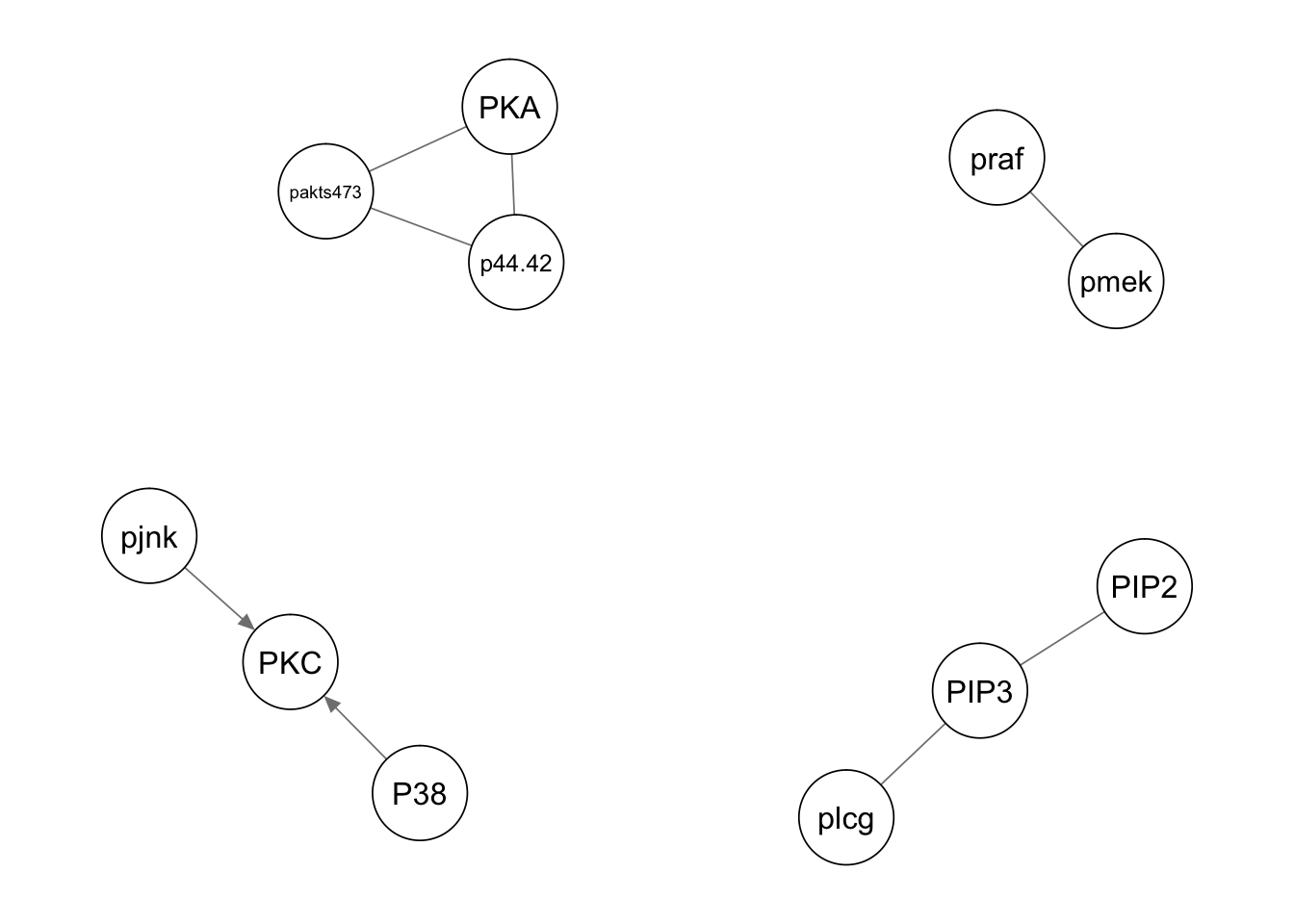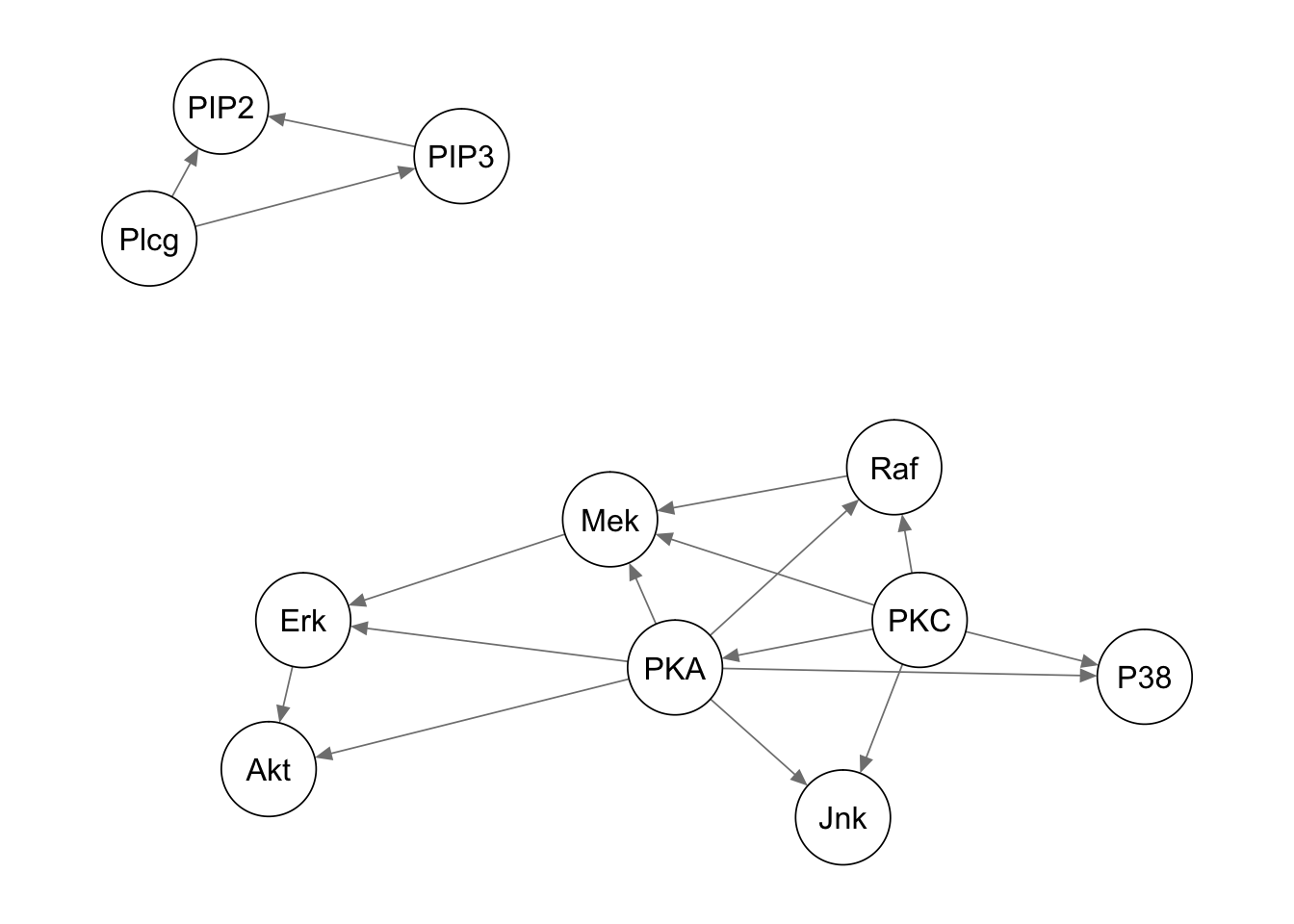## Data

The data consist in the simultaneous measurements of 11 phosphorylated proteins and phospholipids derived from thousands of individual primary immune system cells, subjected to both general and specific molecular interventions (Sachs et al., 2005).

Ref : Scutari, M., & Denis, J. B. (2014). Bayesian networks: with examples in R. CRC press.

``library(bnlearn)``
``````##
## Attaching package: 'bnlearn'``````
``````## The following object is masked from 'package:stats':
##
##     sigma``````
``````library(qgraph)

``````##   praf  pmek  plcg  PIP2  PIP3 p44.42 pakts473 PKA   PKC  P38 pjnk
## 1 26.4 13.20  8.82 18.30 58.80   6.61     17.0 414 17.00 44.9 40.0
## 2 35.9 16.50 12.30 16.80  8.13  18.60     32.5 352  3.37 16.5 61.5
## 3 59.4 44.10 14.60 10.20 13.00  14.90     32.5 403 11.40 31.9 19.5
## 4 73.0 82.80 23.10 13.50  1.29   5.83     11.8 528 13.70 28.6 23.1
## 5 33.7 19.80  5.19  9.73 24.80  21.10     46.1 305  4.66 25.7 81.3
## 6 18.8  3.75 17.60 22.10 10.90  11.90     25.7 610 13.70 49.1 57.8``````

## First DAG

Function gs fit a Gaussian graphical model. By default it fits a directed graph.

``````dag.gs <- gs(sachs, test = "cor")
narcs(dag.gs)``````
``##  8``
``directed.arcs(dag.gs)``
``````##      from   to
## [1,] "P38"  "PKC"
## [2,] "pjnk" "PKC"``````
``qgraph(dag.gs)``This graph can be compared to the one of Sachs. Function model string allows to build a model string from a Bayesian network and vice versa.

``````sachs.modelstring <-
paste("[PKC][PKA|PKC][Raf|PKC:PKA][Mek|PKC:PKA:Raf]",
"[Erk|Mek:PKA][Akt|Erk:PKA][P38|PKC:PKA]",
"[Jnk|PKC:PKA][Plcg][PIP3|Plcg][PIP2|Plcg:PIP3]",sep="")
dag.sachs <- model2network(sachs.modelstring)
qgraph(dag.sachs)``````What are the differences between the two graphs?

# Marginal distributions.

Plot the distributions of Mek, P38, PIP2 and PIP3. Do they agree to the model used to fit the dag.gs?

``# to be filled``

# Dependence between variables

Propose a plot the check if the dependence between the protein concentration can be assumed to be linear.

``# to be filled``

## Discretising Gene Expressions

One way to deal with the non Gaussian assumption as well as the non linearity of the multivaraite distribution is to discetise the gene expressions using information-preserving discretisation. You can use the the discretize function in bnlearn with method = “hartemink”. Hartemink (2001) key idea is to initially discretise each variable into a large number k1 of intervals, thus losing as little information Real-World Applications of Bayesian Networks 145 as possible. Subsequently, the algorithm iterates over the variables and collapses, for each of them, the pair of adjacent intervals that minimises the loss of pairwise mutual information. The algorithm stops when all variables have k2 ≪ k1 intervals left. The resulting set of discretised variables reflects the dependence structure of the original data much better than either quantile or interval discretisation would allow, because the discretisation takes pairwise dependencies into account. Clearly some information is always lost in the process; for instance, higher-level dependencies are completely disregarded and therefore are not likely to be preserved.

``````dsachs <- discretize(sachs, method = "hartemink",
breaks = 3, ibreaks = 60, idisc = "quantile")``````

## Multinomial DAG

Fit a Discrete variable graph using function hc with score = “bde”.

``# to be filled``

## Model averaging

The quality of the structure learned from the data can be improved by averaging multiple CPDAGs.

``````boot <- boot.strength(dsachs, R = 500, algorithm = "hc",
algorithm.args = list(score = "bde", iss = 10))
boot[(boot\$strength > 0.85) & (boot\$direction >= 0.5), ]``````
``````##         from       to strength direction
## 1       praf     pmek    1.000 0.5120000
## 23      plcg     PIP2    0.998 0.5130261
## 24      plcg     PIP3    1.000 0.5260000
## 34      PIP2     PIP3    1.000 0.5120000
## 56    p44.42 pakts473    1.000 0.5730000
## 57    p44.42      PKA    0.988 0.5779352
## 67  pakts473      PKA    1.000 0.5790000
## 89       PKC      P38    1.000 0.5080000
## 90       PKC     pjnk    1.000 0.5110000
## 100      P38     pjnk    0.952 0.5094538``````

Arcs are considered significant if they appear in at least 85% of the networks, and in the direction that appears most frequently. Now let’s average the networks with significant arcs.

``````avg.boot <- averaged.network(boot, threshold = 0.85)
qgraph(avg.boot)``````